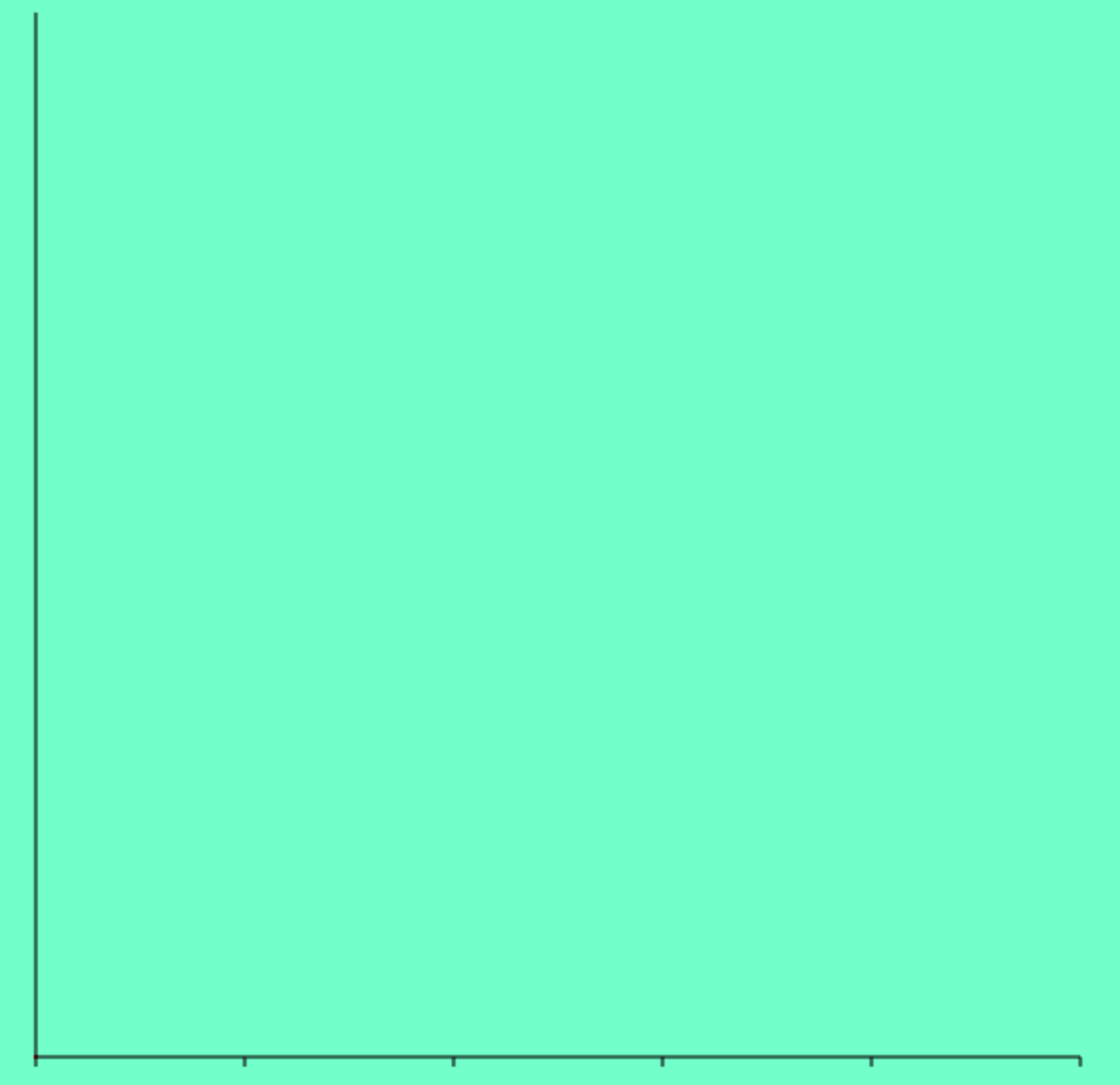墨滴FE-Fly

2021/07/11  阅读：75  主题：凝夜紫

# 前言

1. js实现直线方程
2. 折线图的表达
3. canvas 的一些小技巧

# 直线折线图

1. 直线（两个端点是圆的）
2. 直线（两个端点是直线的）
3. 文字

## 画布的创建

``    class lineChart {        constructor(data, type) {          this.get2d()        }        get2d() {          const canvas = document.getElementById('canvas')          this.ctx = canvas.getContext('2d')        }      }``

``    <style>      * {        padding: 0;        margin: 0;      }      canvas {        background: aquamarine;      }    </style>``

## canvas绘图操作复习

`lineTo(x, y)`

moveTo 是什么就在画布中移动笔触， 也就是你开始画的第一个点，或者你可以想象一下在纸上作业，一支钢笔或者铅笔的笔尖从一个点到另一个点的移动过程。

``moveTo(*x*, *y*)``

``drawtriangle() {  this.ctx.moveTo(25, 25)  this.ctx.lineTo(105, 25)  this.ctx.lineTo(25, 105)}``

1. 首先，你需要创建路径起始点。
2. 然后你使用画图命令去画出路径。
3. 之后你把路径封闭。
4. 一旦路径生成，你就能通过描边或填充路径区域来渲染图形。

``// 通过线条来绘制图形轮廓。ctx.stroke() // 通过填充路径的内容区域生成实心的图形。ctx.fill()``

``this.moveTo(25, 25)this.lineTo(105, 25)this.lineTo(25, 105)this.stroke()``

closePath:

``this.moveTo(25, 25)this.lineTo(105, 25)this.lineTo(25, 105)this.closePath()this.stroke()``

``// 新建一条路径，生成之后，图形绘制命令被指向到路径上生成路径。this.beginPath()``

closePath 其实也不是必须的，如果图形已经是闭合的，就不需要调用， 到这里canvas的基本绘图操作复习就到这里，后面还有一些实战api : 我就例子中给大家讲解， 不然会显得很生硬。

## 封装画直线方法

``export class Point2d {  constructor(x, y) {    this.x = x || 0    this.y = y || 0    this.id = ++current  }  clone() {    return new Point2d(this.x, this.y)  }  equal(v) {    return this.x === v.x && this.y === v.y  }  add2Map() {    pointMap.push(this)    return this  }  add(v) {    this.x += v.x    this.y += v.y    return this  }  abs() {    return [Math.abs(this.x), Math.abs(this.y)]  }  sub(v) {    this.x -= v.x    this.y -= v.y    return this  }  equal(v) {    return this.x === v.x && this.y === v.y  }  rotate(center, angle) {    const c = Math.cos(angle),      s = Math.sin(angle)    const x = this.x - center.x    const y = this.y - center.y    this.x = x * c - y * s + center.x    this.y = x * s + y * c + center.y    return this  }  distance(p) {    const [x, y] = this.clone().sub(p).abs()    return x * x + y * y  }  distanceSq(p) {    const [x, y] = this.clone().sub(p).abs()    return Math.sqrt(x * x + y * y)  }  static random(width, height) {    return new Point2d(Math.random() * width, Math.random() * height)  }  cross(v) {    return this.x * v.y - this.y * v.x  }}``

``new lineChart().drawLine(  Point2d.random(500, 500),  Point2d.random(500, 500))// 画直线drawLine(start, end) {  const { x: startX, y: startY } = start  const { x: endX, y: endY } = end  this.beginPath()  this.moveTo(startX, startY)  this.lineTo(endX, endY)  this.stroke()}``

## js实现直线方程

``````这里没有好展示的，我们还是分析下echarts 官方的折线图直线，直线两旁是两个圆的，想一想？其实这边涉及到一个数学知识，各位小伙伴，Fly再一次化身数学老师给大家讲解，主要是帮有些小伙伴复习复习。  这里我们已经知道直线的开始点和结束点，在数学中我们可以确定一条直线方程，那么我们就可以求出直线上任意一点的（x,y）坐标。那么直线的两个端点的圆心我们就可以确定？ 半径也可以确定了就是圆心分别到开始点和结束点的距离。
``````

1. 一般式： Ax+By+C=0(A、B不同时为0)【适用于所有直线】

2. 点斜式： y-y0=k(x-x0) 【适用于不垂直于x轴的直线 表示斜率为k，且过（x0,y0）的直线

3. 截距式：x/a+y/b=1【适用于不过原点或不垂直于x轴、y轴的直线】

4. 两点式：表示过（x1,y1）和(x2,y2)的直线　【适用于不垂直于x轴、y轴的直线】 (x1≠x2，y1≠y2)

``export function computeLine(p0, p1, t) {  let x1 = p0.x  let y1 = p0.y  let x2 = p1.x  let y2 = p1.y  // 说明直线平行 y轴  if (x1 === x2) {    return new Point2d(x1, t)  }  // 平行X轴的情况  if (y1 === y2) {    return new Point2d(t, y1)  }  const y = ((t - x1) / (x2 - x1)) * (y2 - y1) + y1  return new Point2d(t, y)}``

p0、p1、 对应的两个直线点 t 就是参数，对应直线的x,我们求出y，返回新的点就好了 。我们默认以开始点和结束点的 x 位置分别 减去或者加一个固定的值 ， 求得圆心。直接看下图吧：

``arc(x, y, radius, startAngle, endAngle, anticlockwise)``

``drawCircle(center, radius = 4) {  const { x, y } = center  this.ctx.beginPath()  this.ctx.arc(x, y, radius, 0, Math.PI * 2, true) // 绘制  this.ctx.fill()}``

1. 先画开始圆
2. 画直线
3. 画结束圆

``drawLineCircle(start, end, type) {  const flag = type === 'left'  const { x: startX, y: startY } = start  const { x: endX, y: endY } = end  const center = this.getOnePointOnLine(    start.clone(),    end.clone(),    flag ? startX - this.distance : endX + this.distance  )  // 两点之间的距离  不熟悉的小伙伴可以看下上面的文章  const radius = (flag ? start : end).clone().distanceSq(center)  this.drawCircle(center, radius)}``

## 画XY坐标轴

​ 坐标轴本质上就是两条直线，所以第一步确定坐标原点，然后以坐标原点画出垂直和水平的两条直线。 我们设置坐标原点离画布的左内边距和底部内边距，这样我们可以通过画布的高度减去底部内边距得到 原点的y, 然后通过画布的宽度减去左内边距得到x, 有了坐标原点画坐标轴就没什么大问题了。代码如下：

``  //定义坐标轴相对于画布的内边距  this.paddingLeft = 30 // 至少大于绘制文字的宽度  this.paddingBottom = 30 // 至少大于绘制文字的高度  this.origin = new Point2d(    this.paddingLeft,    this.height - this.paddingBottom  )  this.drawCircle(this.origin, 1, 'red')  this.addxAxis()  this.addyAxis()  // 画 x 轴  addxAxis() {    const end = this.origin      .clone()      .add(new Point2d(this.width - this.paddingLeft * 2, 0))    this.drawLine(this.origin, end)  }    // 画y轴  addyAxis() {    const end = this.origin      .clone()      .sub(new Point2d(0, this.height - this.paddingBottom * 2))    this.drawLine(this.origin, end)  }``

`` drawLineWithDiscrete(start, end, n = 5) {    // 由于 x 轴上的 y 都是相同的    const points = []    const startX = start.x    const endX = end.x    points.push(start)    const segmentValue = (endX - startX) / n    for (let i = 1; i <= n - 1; i++) {      points.push(new Point2d(startX + i * segmentValue, start.y))    }    points.push(end)    // 生成线段    points.forEach((point) => {      this.drawLine(point, point.clone().add(new Point2d(0, 5)))    })  }``这时候还差文字，canvas 绘制文字的api

``在指定的(x,y)位置填充指定的文本，绘制的最大宽度是可选的.ctx.fillText（text,x,y,[,maxwidth]）``

`` this.axisData = ['Mon', 'Tue', 'Wed', 'Thu', 'Fri', 'Sat', 'Sun'] this.yxisData = ['0', '50', '100', '150', '200', '250', '300']``

``// 生成X轴文字的点const segmentValue = (endX - startX) / nfor (let i = 0; i <= n - 1; i++) {  const textpoint = new Point2d(    startX + i * segmentValue + segmentValue / 2,    start.y + 20  )  // 这里每个点的文字与X轴数据是互相呼应的  textPoints.push({    point: textpoint,    text: this.axisData[i],  })}// 生成文字this.clearFillColor()textPoints.forEach((info) => {  const { text, point } = info  this.ctx.fillText(text, point.x, point.y)})``

``// 限制文字的长度this.ctx.fillText(text, point.x, point.y, 20)// 文字的每个点要减去长度的一半const textpoint = new Point2d(  startX + i * segmentValue + segmentValue / 2 - 10,  start.y + 20)``

X轴的处理好了，我们处理Y轴，Y轴其实相对比较简单就是每个数据对应的一条直线。

Y轴的话也是要计算每个线段的长度的值，然后画出直线， 这里要特别注意的是就是文字的放置， 在每个端点还要进行微调。使得文字和直线居中对齐。代码如下：

``addyAxis() {  const end = this.origin    .clone()    .sub(new Point2d(0, this.height - this.paddingBottom * 2))  const points = []  const length = this.origin.y - end.y  const segmentValue = length / this.yxisData.length  for (let i = 0; i < this.yxisData.length; i++) {    const point = new Point2d(end.x, this.origin.y - i * segmentValue)    points.push({      point,      text: this.yxisData[i],    })  }  points.forEach((info) => {    const { text, point } = info    const end = point      .clone()      .add(new Point2d(this.width - this.paddingLeft * 2, 0))    this.setStrokeColor('#E0E6F1')    this.drawLine(point, end)    this.clearStrokeColor()    this.ctx.fillText(text, point.clone().x - 30, point.y + 4, 20)  })}``

``const length = this.origin.y - end.yconst division = length / 300const point = new Point2d(end.x, this.origin.y - i * division * 50)``

``this.realData = [150, 230, 224, 218, 135, 147, 260]this.xPoints = []this.yPoints = []``

``// 生成文字this.clearFillColor()textPoints.forEach((info) => {  const { text, point } = info  this.xPoints.push(point.x)  this.ctx.fillText(text, point.x, point.y, 20)})``

yPoints其实也就比较简单了， 真实数据 * 每一份的距离就好了。

``const division = length / 300for (let i = 0; i < this.yxisData.length; i++) {  const point = new Point2d(end.x, this.origin.y - i * division * 50)  // 在这里， 还是得注意坐标轴的位置   const realData = this.realData[i]  this.yPoints.push(this.origin.y - realData * division)  points.push({    point,    text: this.yxisData[i],  })}``

``let start = new Point2d(this.xPoints, this.yPoints)// 生成折线图this.setStrokeColor('#5370C6')this.xPoints.slice(1).forEach((x, index) => {  const end = new Point2d(x, this.yPoints[index + 1])  this.drawLineWithCircle(start, end)  start = end})``

1. 存在的圆点重复
2. 圆点的半径大小不一致，说明我们之前计算圆心到直线的距离 这样设为 半径是错误的， 因为每条的线的斜率是不一样的。所以算出来是有问题的。

``let start = new Point2d(this.xPoints, this.yPoints)this.drawCircle(start)// 生成折线图this.setStrokeColor('#5370C6')this.xPoints.slice(1).forEach((x, index) => {  const end = new Point2d(x, this.yPoints[index + 1])  // 画圆  this.drawCircle(end)  // 画直线  this.drawLine(start, end)  start = end})``

# 显示tooltip

``#tooltip {  position: absolute;  z-index: 2;  background: white;  padding: 10px;  border-radius: 2px;  visibility: hidden;}<div id="tooltip"></div>``

``canvas.addEventListener('mousemove', this.onMouseMove.bind(this))// 这里取相对于画布原点的位置 offset onMouseMove(e) {  const x = e.offsetX  const y = e.offsetY}``

``onMouseMove(e) {  const x = e.offsetX  const find = this.xPoints.findIndex(    (item) => Math.abs(x - item) <= this.tolerance  )  if (find > -1) {    this.tooltip.textContent = `数据：\${this.axisData[find]}_ \${this.yxisData[find]}`    this.tooltip.style.visibility = 'visible'    this.tooltip.style.left = e.clientX + 2 + 'px'    this.tooltip.style.top = e.clientY + 2 + 'px'  } else {    this.tooltip.style.visibility = 'hidden'  }}``

## 画垂直的虚线

``drawDashLine(start, end) {  if (!start || !end) {    return  }  this.ctx.setLineDash([5, 10])  this.beginPath()  this.moveTo(start.x, start.y)  this.lineTo(end.x, end.y)  ctx.stroke()}``

``onMouseMove(e) {  const x = e.offsetX  const find = this.xPoints.findIndex(    (item) => Math.abs(x - item) <= this.tolerance  )  if (find > -1) {    this.tooltip.textContent = `数据：\${this.axisData[find]}_ \${this.yxisData[find]}`    this.tooltip.style.visibility = 'visible'    this.tooltip.style.left = e.clientX + 2 + 'px'    this.tooltip.style.top = e.clientY + 2 + 'px'    // 画虚线    const start = new Point2d(this.xPoints[find], this.origin.y)    const end = new Point2d(this.xPoints[find], 0)    this.drawDashLine(start, end)  } else {    this.tooltip.style.visibility = 'hidden'  }}``

``clearData() {  this.ctx.clearRect(0, 0, 600, 600)  this.xPoints = []  this.yPoints = []}``

``const start = new Point2d(this.xPoints[find], this.origin.y)const end = new Point2d(this.xPoints[find], 0)// 清除数据this.clearData()this.drawDashLine(start, end)// 虚线样式也要每次清除 不然会影响下面的画的样式this.ctx.setLineDash([])this.addxAxis()this.addyAxis()this.setStrokeColor('#5370C6')this.generateLineChart()``

## restore和save的妙用

``drawDashLine(start, end) {    if (!start || !end) {      return    }    this.ctx.save()    this.ctx.setLineDash([5, 10])    this.beginPath()    this.moveTo(start.x, start.y)    this.lineTo(end.x, end.y)    this.stroke()    this.ctx.restore()  }``

# 源码下载FE-Fly

2021/07/11  阅读：75  主题：凝夜紫